# Profit and Loss – Concepts and Formula

## Profit and Loss – Concepts and Formula

‘Profit and loss’ is one of several application-based topic that deal with everyday transactions in one’s life. When things are acquired from a seller and then sold to a buyer, profit and loss occur. In this article, we’ll go through all of the profit and loss concepts and formulas. The following are some key concepts and formulas. relating to profit and loss. Before you begin solving profit and loss cases, you must first understand these concepts.

Cost Price: The price at which an article is purchased, is called its cost price, abbreviated as C.P.

Selling Price: The price at which an article is sold, is called its selling price, abbreviated as S.P.
Case – I If selling price is more than cost price, then the seller is in profit.

Selling Price = Cost Price + Profit

Case-II If the cost price is more than selling price, then the seller incurred loss.

Selling Price = Cost Price – loss

Profit or Gain: If S.P. is greater than C.P., the seller is said to have a profit or gain.

Profit = S.P – C.PQuestion. Mansi purchased a bag for Rs 2,500 and sold it for Rs 3,000. What is the profit and profit percent she made on the bag?
Answer. Here CP = 2,500 and SP = 3,000
Profit = S.P – C.P = 3,000 – 2,500 = 500Loss: If S.P. is less than C.P., the seller is said to have incurred a loss.

Loss = C.P – S.PQuestion. Lokesh purchased a Pen for Rs 30 and sold it for Rs 25 after two days. What is the loss and loss percent he made on the Pen?
Answer. Here CP = 30 and SP = 25
Loss = C.P – S.P = 30 – 25 = 5Note : Profit % and loss % always calculated on C.P

• When we want to calculate the S.P and we know the C.P and Profit/Loss %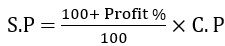We can say that SP is (100+x)% of CP if there is x% profit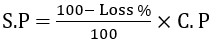We can say that SP is (100 – x )% of CP if there is x% loss

Question. Find S.P if C.P = 55 and Profit % = 20%.
Answer. Profit = 20% of 55 = 11
S.P = C.P + Profit = 55 + 11 = 66, or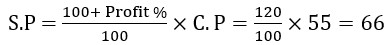In this case, SP is 120% of CP as there is 20% profit

Question. Find S.P if C.P = 45 and Loss % = 20%.
Answer. In this case SP is (100-20)% of CP as there is 20% loss
SP = 80% of 45 = 36, or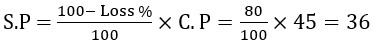Note: We do not have to remember the formula for this,

• If an article is sold at a gain of say, 25%, then S.P. = 125% of C.P.
• If an article is sold at a loss of say, 15%, then S.P. = 85% of C.P.

• When we want to calculate the C.P and we know the S.P and Profit/Loss %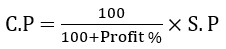&Question. Find C.P if S.P = 60 and Profit % = 20%.
Answer. Let CP be x
Profit + CP = SP
20% of x + x = 60
0.2x + x = 60
1.2x = 60
x = 60/1.2 = 50, or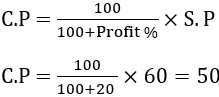Question Vandana sold a mobile phone at the cost of Rs 1800 at a loss of 25%. At what cost will she have to sell it to get a profit of 30%?
Case I.  S.P. = 1800, Loss = 25%.
75% of C.P = S.P
75/100 x C.P = 1800
C.P = 1800 x 100/75 = 2400, orCase II.  C.P. = 2400, Profit = 30%.
S.P = 130% of CP
S.P = 130/100 x 2400 = 3120

Read More Quantitative Aptitude concepts

#### Aatma Nirbhar Reasoning Topic Wise Online Test Series

Recommended PDF’s for :

#### Most important PDF’s for Bank, SSC, Railway and Other Government Exam : Download PDF Now

AATMA-NIRBHAR Series- Static GK/Awareness Practice Ebook PDF Get PDF here
The Banking Awareness 500 MCQs E-book| Bilingual (Hindi + English) Get PDF here
AATMA-NIRBHAR Series- Banking Awareness Practice Ebook PDF Get PDF here
Computer Awareness Capsule 2.O Get PDF here
AATMA-NIRBHAR Series Quantitative Aptitude Topic-Wise PDF Get PDF here
AATMA-NIRBHAR Series Reasoning Topic-Wise PDF Get PDF Here
Memory Based Puzzle E-book | 2016-19 Exams Covered Get PDF here
Caselet Data Interpretation 200 Questions Get PDF here
Puzzle & Seating Arrangement E-Book for BANK PO MAINS (Vol-1) Get PDF here
ARITHMETIC DATA INTERPRETATION 2.O E-book Get PDF here
3